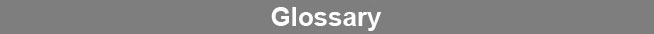Select Page### Coefficient of Variation

Coefficient of variation (CV) is a statistical measurement of the distribution of responses around the mean of those values. The CV is applicable only to those values that come from a Gaussian distributed population. It is derived by dividing the standard deviation of the replicate adjusted or normalized response measurements by the mean of the replicate measurements, multiplied by 100 for percent. Since the measurement units (e.g., CPM) are canceled out, the %CV is a relative value that is independent of the magnitude of replicate response measurements.www.brendan.com